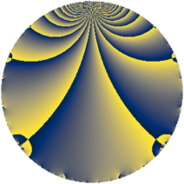# Properties

 Label 370.2.aLevel $370$ Weight $2$ Character orbit 370.a Rep. character $\chi_{370}(1,\cdot)$ Character field $\Q$ Dimension $11$ Newform subspaces $7$ Sturm bound $114$ Trace bound $3$

# Related objects

## Defining parameters

 Level: $$N$$ $$=$$ $$370 = 2 \cdot 5 \cdot 37$$ Weight: $$k$$ $$=$$ $$2$$ Character orbit: $$[\chi]$$ $$=$$ 370.a (trivial) Character field: $$\Q$$ Newform subspaces: $$7$$ Sturm bound: $$114$$ Trace bound: $$3$$ Distinguishing $$T_p$$: $$3$$, $$7$$

## Dimensions

The following table gives the dimensions of various subspaces of $$M_{2}(\Gamma_0(370))$$.

Total New Old
Modular forms 60 11 49
Cusp forms 53 11 42
Eisenstein series 7 0 7

The following table gives the dimensions of the cuspidal new subspaces with specified eigenvalues for the Atkin-Lehner operators and the Fricke involution.

$$2$$$$5$$$$37$$FrickeDim
$$+$$$$+$$$$+$$$+$$$1$$
$$+$$$$+$$$$-$$$-$$$1$$
$$+$$$$-$$$$+$$$-$$$1$$
$$+$$$$-$$$$-$$$+$$$2$$
$$-$$$$+$$$$+$$$-$$$3$$
$$-$$$$-$$$$-$$$-$$$3$$
Plus space$$+$$$$3$$
Minus space$$-$$$$8$$

## Trace form

 $$11 q + q^{2} + 11 q^{4} + q^{5} + 4 q^{6} - 8 q^{7} + q^{8} + 15 q^{9} + O(q^{10})$$ $$11 q + q^{2} + 11 q^{4} + q^{5} + 4 q^{6} - 8 q^{7} + q^{8} + 15 q^{9} - q^{10} + 8 q^{11} - 2 q^{13} + 4 q^{14} + 4 q^{15} + 11 q^{16} + 10 q^{17} + 13 q^{18} - 8 q^{19} + q^{20} + 12 q^{22} - 8 q^{23} + 4 q^{24} + 11 q^{25} + 10 q^{26} - 24 q^{27} - 8 q^{28} - 10 q^{29} - 16 q^{31} + q^{32} - 24 q^{33} - 6 q^{34} - 4 q^{35} + 15 q^{36} + q^{37} + 4 q^{38} - 40 q^{39} - q^{40} - 14 q^{41} - 8 q^{42} - 4 q^{43} + 8 q^{44} - 3 q^{45} + 8 q^{46} - 8 q^{47} - 9 q^{49} + q^{50} - 2 q^{52} + 38 q^{53} - 8 q^{54} - 12 q^{55} + 4 q^{56} + 8 q^{57} + 10 q^{58} + 16 q^{59} + 4 q^{60} - 10 q^{61} - 20 q^{62} - 32 q^{63} + 11 q^{64} + 2 q^{65} - 8 q^{66} + 10 q^{68} - 16 q^{69} + 4 q^{70} + 16 q^{71} + 13 q^{72} - 2 q^{73} - q^{74} - 8 q^{76} + 16 q^{77} - 40 q^{78} - 24 q^{79} + q^{80} + 3 q^{81} - 6 q^{82} - 24 q^{83} + 6 q^{85} - 8 q^{86} - 24 q^{87} + 12 q^{88} + 6 q^{89} - 13 q^{90} - 16 q^{91} - 8 q^{92} - 16 q^{93} - 12 q^{94} - 4 q^{95} + 4 q^{96} + 10 q^{97} + 9 q^{98} + 56 q^{99} + O(q^{100})$$

## Decomposition of $$S_{2}^{\mathrm{new}}(\Gamma_0(370))$$ into newform subspaces

Label Dim $A$ Field CM Traces A-L signs $q$-expansion
$a_{2}$ $a_{3}$ $a_{5}$ $a_{7}$ 2 5 37
370.2.a.a $1$ $2.954$ $$\Q$$ None $$-1$$ $$-2$$ $$-1$$ $$-1$$ $+$ $+$ $-$ $$q-q^{2}-2q^{3}+q^{4}-q^{5}+2q^{6}-q^{7}+\cdots$$
370.2.a.b $1$ $2.954$ $$\Q$$ None $$-1$$ $$0$$ $$-1$$ $$0$$ $+$ $+$ $+$ $$q-q^{2}+q^{4}-q^{5}-q^{8}-3q^{9}+q^{10}+\cdots$$
370.2.a.c $1$ $2.954$ $$\Q$$ None $$-1$$ $$2$$ $$1$$ $$1$$ $+$ $-$ $+$ $$q-q^{2}+2q^{3}+q^{4}+q^{5}-2q^{6}+q^{7}+\cdots$$
370.2.a.d $1$ $2.954$ $$\Q$$ None $$1$$ $$-2$$ $$1$$ $$2$$ $-$ $-$ $-$ $$q+q^{2}-2q^{3}+q^{4}+q^{5}-2q^{6}+2q^{7}+\cdots$$
370.2.a.e $2$ $2.954$ $$\Q(\sqrt{3})$$ None $$-2$$ $$-2$$ $$2$$ $$-6$$ $+$ $-$ $-$ $$q-q^{2}+(-1+\beta )q^{3}+q^{4}+q^{5}+(1+\cdots)q^{6}+\cdots$$
370.2.a.f $2$ $2.954$ $$\Q(\sqrt{33})$$ None $$2$$ $$4$$ $$2$$ $$-3$$ $-$ $-$ $-$ $$q+q^{2}+2q^{3}+q^{4}+q^{5}+2q^{6}+(-1+\cdots)q^{7}+\cdots$$
370.2.a.g $3$ $2.954$ 3.3.892.1 None $$3$$ $$0$$ $$-3$$ $$-1$$ $-$ $+$ $+$ $$q+q^{2}-\beta _{2}q^{3}+q^{4}-q^{5}-\beta _{2}q^{6}+\cdots$$

## Decomposition of $$S_{2}^{\mathrm{old}}(\Gamma_0(370))$$ into lower level spaces

$$S_{2}^{\mathrm{old}}(\Gamma_0(370)) \cong$$ $$S_{2}^{\mathrm{new}}(\Gamma_0(37))$$$$^{\oplus 4}$$$$\oplus$$$$S_{2}^{\mathrm{new}}(\Gamma_0(74))$$$$^{\oplus 2}$$$$\oplus$$$$S_{2}^{\mathrm{new}}(\Gamma_0(185))$$$$^{\oplus 2}$$